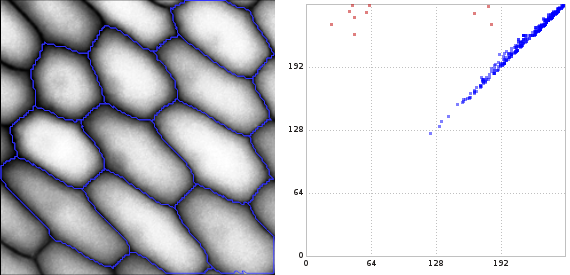# libstick

Libstick is a C++ library to compute persistent homology for abstract simplicial complexes over $$\mathbb{Z}_2$$. It basically implements the boundary matrix reduction algorithm as given in Edelsbrunner and Harer1. Its main features are:

• It computes the reduced boundary matrix and the transformation matrix from the boundary matrix of a given simplicial complex. The former gives rise to the persistence diagram or the persistence barcode. The latter gives you (a cycle of) the actual homology classes that are born and die through the filtration.

• It is algorithmically simple and has less than 2000 lines of code.

• It is implemented as template classes. The dimension of simplicial complexes or the index type of the simplices are given as template parameters.

• To speed up computation, the sparse Boolean column vectors of the involved matrices are implemented as sorted index vectors rather than linked lists.

On my X220 Thinkpad, computing the reduced boundary matrix, the transformation matrix and the persistence diagrams takes about 1.5 seconds for 1 Million simplices originating from a random 2D image. However, if you are looking for a persistent homology library optimized for performance, I refer to PHAT and DIPHA.

• Libstick may be useful for students or teachers to illustrate persistence on real-world examples. For instance, it allows to print information on the sequence on births and deaths of cycles during the sequence of simplex insertions during the reduction algorithm.

## Installation

See the installation instructions.

## Getting started

The getting started page gives a very brief introduction into the notion of persistent homology and how these concepts are mapped to libstick. If you are familiar with persistent homology then probably the picture below tells most of the story already:## Demonstration

The demonstration page page contains two simple toy applications of persistent homology in image processing.1. H. Edelsbrunner and J. Harer, Computational Topology. An Introduction, 2010, ISBN 0-8218-4925-5.